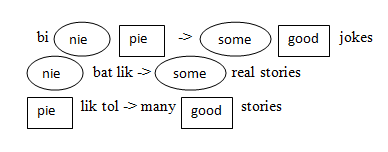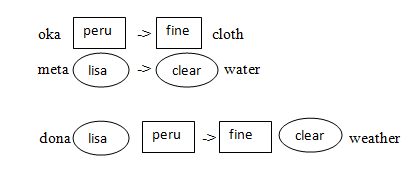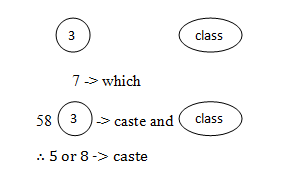# Coding and Decoding ( 21 to 25 )

#### PREVNEXT

Coding and Decoding ( 21 to 25 ):

# APTITUDE QUESTIONS (CODING AND DECODING):

21. In a certain code ‘bi nie pie’ means ‘some good jokes’, ‘nie bat lik’ means ‘some real stories’, and ‘pie lik tol’ means ‘many good stories’. Which word in that code means ‘jokes’?

A) Bi

B) Nie

C) Pie

D) Lik

E) None of these

Explanation:bi means jokes

22. If in a certain language, ‘oka peru’ means ‘fine cloth’, ‘meta lisa’ means ‘clear water’ and ‘dona lisa peru’ means ‘fine clear weather’, then which word in that language means ‘weather’?

A) Peru

B) oka

C) meta

D) dona

E) None of these

Explanation:23. In a certain code, ‘37’ means ‘which class’ and ‘583’ means ‘caste and class’. What is the code for ‘caste’?

A) 3

B) 7

C) 8

D) Either 5 or 8

E) None of these

Explanation:24. If DELHI is coded as 73541 and CALCUTTA as 82589662, how will CALICUT be coded?

A) 5729431

B) 5798213

C) 8251896

D) 8543691

E) None of these

Explanation:

DELHI

D = 7, E = 3, L = 5, H = 4, I = 1

CALCUTTA

C = 8, A = 2, L = 5, C = 8, U = 9, T = 6, T = 6, A = 2

∴ CALICUT = 8251896

25. If SHARP is coded as 58034 and PUSH is coded as 4658, then RUSH is coded as:

A) 5729431

B) 5798213

C) 8251896

D) 8543691

E) None of these

Explanation:

SHARP

S = 5, H = 8, A = 0, R = 3, P = 4

PUSH

P = 4, U = 6. S = 5, H = 8

So, in the same way

RUSH = 3658